Author: Oscar Cronquist Article last updated on November 23, 2021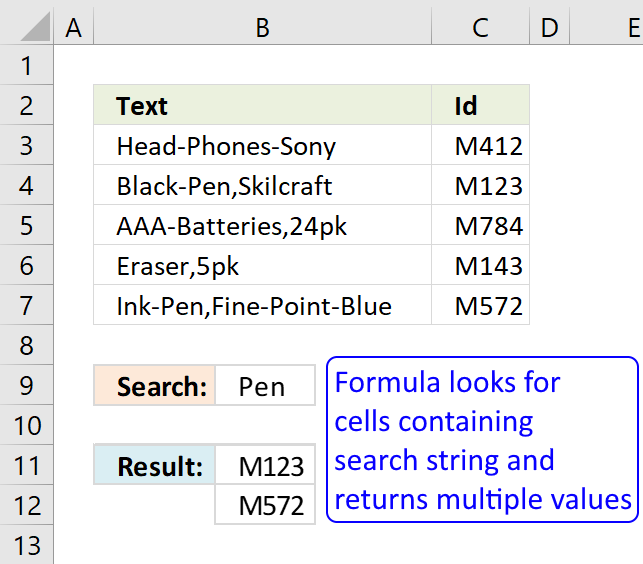This article demonstrates array formulas that search for cell values containing a search string and returns corresponding values on the same row.

The functions used in most of the formulas on this web page are the SEARCH and FIND functions. They return a number based on the position of a text string in a value, see cell E3 on picture above. If the text string is not found the functions return #VALUE! error.

"fox" is found at character 7 in value "a red fox", see picture below.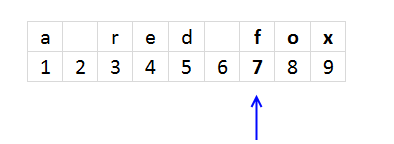The SEARCH function is case-insensitive and the FIND function is case-sensitive. You can replace these functions with each other if you are looking for a case-sensitive formula or vice versa.

The magic starts when you enter the formula as an array formula, this allows you to search an entire cell range for text strings.

### Search for a substring in a column and return multiple corresponding values

I need a formula with no Macros – here an example of what I’m trying to do.

Column A contains:
Black-Pen,Skilcraft
AAA-Batteries,24pk
Eraser,5pk
Ink-Pen,Fine-Point-Blue

Column B contains:

M412
M123
M784
M143
M572

In Cell D1 I want to ENTER *Pen* and have it list all corresponding values which is Cell A2 & Cell A5

It sounds like a “Lookup one value with multiple corresponding values” but when I use a wildcard in my search it doesn’t work... Do you have a solution for it? Thank you

The picture below shows an array formula in cell F4 that searches cell range B3:B7 for the text string in cell F2 "Pen" and returns the adjacent value, on the same row, from column C.

Text string "Pen" is found in cell B4 and B7 so the formula returns adjacent value M123 and M572 to cell range F4:F5.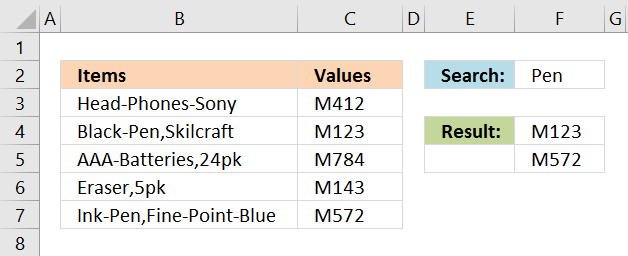Array formula in cell F4:

=INDEX(\$C\$3:\$C\$7, SMALL(IF(ISNUMBER(SEARCH(\$F\$2, \$B\$3:\$B\$7)), MATCH(ROW(\$B\$3:\$B\$7), ROW(\$B\$3:\$B\$7))), ROWS(\$A\$1:A1)))

#### Watch a video where I explain the formula above

Update 1/12/2021 - new dynamic array formula

Dynamic array formula in cell F4:

=FILTER(\$C\$3:\$C\$7, ISNUMBER(SEARCH(\$F\$2, \$B\$3:\$B\$7)))

This formula contains the new FILTER function and works only for Excel 365 subscribers.

The following article explains how to look for values that contain two different text strings:

Recommended articles

Partial match for multiple strings – AND logic
This article demonstrates formulas that let you perform partial matches based on multiple strings and return those strings if all […]

This post explains how to look for strings in a cell value and return multiple corresponding values:

Recommended articles

Return multiple matches with wildcard vlookup
Mr.Excel had a "challenge of the month" June/July 2008 about Wildcard VLOOKUP: "The data in column A contains a series […]

#### How to enter an array formula

1. Copy formula
2. Select cell E2
3. Paste formula
4. Press and hold Ctrl + Shift
5. Press Enter

Recommended article

Recommended articles

A beginners guide to Excel array formulas
Array formulas allows you to do advanced calculations not possible with regular formulas.

#### How to copy array formula

1. Copy cell E2
2. Paste to cell range E3:E4

#### Explaining array formula in cell E2

Step 1 - Search for a specific text string

SEARCH(\$E\$1, \$A\$1:\$A\$5)

becomes

SEARCH("Pen", {"Head-Phones-Sony"; "Black-Pen,Skilcraft"; "AAA-Batteries,24pk"; "Eraser,5pk"; "Ink-Pen,Fine-Point-Blue"})

and returns

{#VALUE!;7;#VALUE!;#VALUE!;5}

Recommended articles

How to use the SEARCH function
Returns a number representing the position a substring is, if found in a textstring. FIND is case sensitive and SEARCH is not.

Step 2 - Check if  values in array contains a a number

ISNUMBER(SEARCH(\$E\$1, \$A\$1:\$A\$5))

becomes

ISNUMBER({#VALUE!;7;#VALUE!;#VALUE!;5})

and returns

{FALSE; TRUE; FALSE; FALSE; TRUE}

Step 3 - Convert number to a row number

IF(ISNUMBER(SEARCH(\$E\$1, \$A\$1:\$A\$5)), MATCH(ROW(\$A\$1:\$A\$5), ROW(\$A\$1:\$A\$5)))

becomes

IF({FALSE; TRUE; FALSE; FALSE; TRUE}, MATCH(ROW(\$A\$1:\$A\$5), ROW(\$A\$1:\$A\$5)))

becomes

IF({FALSE; TRUE; FALSE; FALSE; TRUE}, {1; 2; 3; 4; 5})

and returns

{FALSE; 2; FALSE; FALSE; 5}

Recommended articles

How to use the IF function
Checks if a logical expression is met. Returns a specific value if TRUE and another specific value if FALSE.

Step 5 - Filter n-th smallest row number in array

SMALL(IF(ISNUMBER(SEARCH(\$E\$1, \$A\$1:\$A\$5)), MATCH(ROW(\$A\$1:\$A\$5), ROW(\$A\$1:\$A\$5))), ROW(A1))

becomes

SMALL({FALSE; 2; FALSE; FALSE; 5}, ROW(A1))

becomes

SMALL({FALSE; 2; FALSE; FALSE; 5}, 1)

and returns 2.

Recommended articles

How to use the SMALL function
The SMALL function lets you extract a number in a cell range based on how small it is compared to the other numbers in the group.

Step 6 - Return adjacent value

INDEX(\$B\$1:\$B\$5, SMALL(IF(ISNUMBER(SEARCH(\$E\$1, \$A\$1:\$A\$5)), MATCH(ROW(\$A\$1:\$A\$5), ROW(\$A\$1:\$A\$5))), ROW(A1)))

becomes

INDEX(\$B\$1:\$B\$5, 2)

becomes

INDEX({"M412";"M123";"M784";"M143";"M572"}, 2)

and returns "M123" in cell E2.

Recommended articles

How to use the INDEX function
Gets a value in a specific cell range based on a row and column number.

### Get the Excel file### Search for a text string in a column and return multiple adjacent values corresponding to the number of matching values found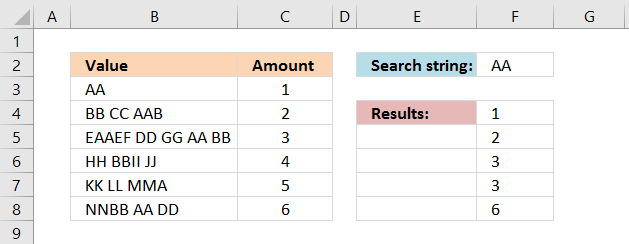The picture above demonstrates an array formula that searches a cell range for a text string and returns the corresponding value on the same row as many times as the textstring is found in the value.

Example, cell B5 has value "EAAEF DD GG AA BB". Text string "AA" is found twice so the corresponding value on the same row is also returned twice (3).

Array formula in cell F4:

=INDEX(\$C\$3:\$C\$8, SMALL(IF(COUNTIF(\$F\$3:F3, \$C\$3:\$C\$8)<>(LEN(\$B\$3:\$B\$8)-LEN(SUBSTITUTE(\$B\$3:\$B\$8, \$F\$2, "")))/LEN(\$F\$2), MATCH(ROW(\$B\$3:\$B\$8), ROW(\$B\$3:\$B\$8)), ""), 1))

### Get the Excel fileSearch for a text string in a column and return multiple adjacent values corresponding to the number of matching values found.xlsx

### Search for multiple text strings in a column and return multiple adjacent values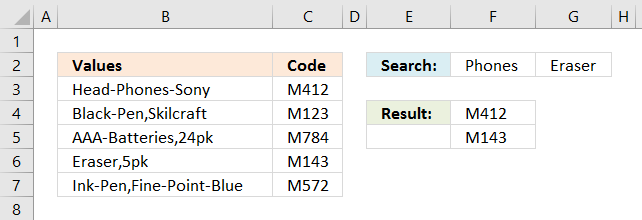This array formula searches for both "Phones" and "Eraser" in column B, if at least one of them is found the corresponding value in column C is returned to F4 and F5.

Array formula in cell F4:

=INDEX(\$C\$1:\$C\$5, SMALL(IF(ISNUMBER(SEARCH(\$F\$1:\$G\$1, \$A\$1:\$B\$5)), MATCH(ROW(\$B\$1:\$B\$5), ROW(\$B\$1:\$B\$5))), ROWS(\$A\$1:A1)))

You can have more than two search strings if you like however they must be arranged horizontally (on the same row). If you want the search strings vertically (on the same column) use the TRANSPOSE function, like this: TRANSPOSE(\$F\$1:\$F\$2)

How to enter an array formula

Update 1/12/2021 - new dynamic array formula

Dynamic array formula in cell F4:

=FILTER(C1:C5, ISNUMBER(SEARCH(\$F\$1, \$B\$1:\$B\$5))+ISNUMBER(SEARCH(\$G\$1, \$B\$1:\$B\$5)))

This formula contains the new FILTER function and works only for Excel 365 subscribers.

### Get the Excel fileSearch for multiple text strings in a column and return multiple adjacent values.xlsx

### Search for a text string in multiple columns and return adjacent values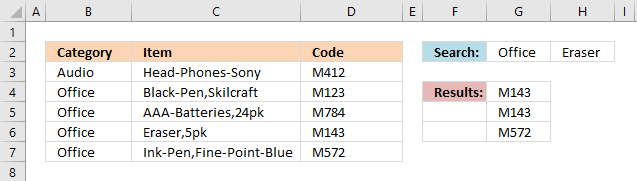The picture above shows two search text strings in cell range G2:H2, the array formula in cell range G4:G7 searches in both columns B and C. If at least one text string is found the corresponding value in column D on the same row is returned to G4:G7.

Array formula in cell G4:

=INDEX(\$C\$1:\$C\$5, SMALL(IF(ISNUMBER(SEARCH(\$F\$1:\$G\$1, \$A\$1:\$B\$5)), MATCH(ROW(\$B\$1:\$B\$5), ROW(\$B\$1:\$B\$5))), ROWS(\$A\$1:A1)))

You can have more than two search strings if you like, however, they must be arranged horizontally (on the same row). If you want the search strings vertically (on the same column) use the TRANSPOSE function, like this: TRANSPOSE(\$G\$1:\$G\$2)